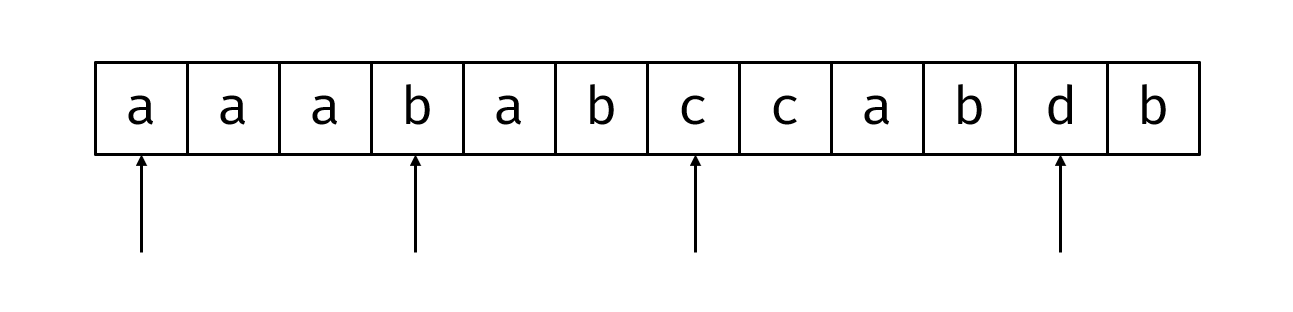# 分析

$$\begin{eqnarray} g(k) & = & \sum_{i = k}^n a_i \frac{i!}{k!(i-k)!} \ g(k) & = & \frac{1}{k!} \sum_{i = k}^{n} (a_i \cdot i!) \times \frac{1}{(i-k)!} \end{eqnarray}$$

rank solved A B C D E F G H I J K L
4 7 O . O O . . O O O O Ø .

# 分析rank solved A B C D E F G H I J K L M
54 9 Ø Ø . Ø O . O . O Ø O . Ø

## 题面

1. 点 $u$ 下班，加入栈中；

2. 栈顶 $u$ 弹栈，$u$ 重新上班；

3. 报告点 $u$ 处于满足状态。

# G Clusterization Counting

rank solved A B C D E F G H I J K
115 6 Ø O Ø Ø O . O O O Ø O